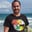Related Tags

python
communitycreator

# How to find the mean, median, and mode in PythonChris Zichko

Hello there, we will start this shots off with an overview of the statistics module in Python.

The version of Python I will be demonstrating is Python 3.8.

The statistics module is essential to learn since most Python programmers are in, or interested in, the Machine Learning field. So, it would be safe to assume that this information will be used at some point.

## Time to dive into the depths of this module.

According to the Merriam-Webster dictionary, the definition of statistics is, “a branch of mathematics dealing with the collection, analysis, interpretation, and presentation of masses of numerical data.” In other words, the statistics module has taken all the mathematics inside the statistics branch of mathematics and translated it with Python, so the computer understands it.

I know that doesn’t seem very interesting, but this can be a handy tool depending on the project. For example, when you get massive files containing a lot of data, you can find the average and central locations of data using simple statistical mathematics like mean, median, and mode.

You can also use this module to find any deviations in data, typically called the “measures of spread.” Deviant data is data that is too far removed from the average, or mean, of the data set to be considered valid values in the data set.

Alright, time for some coding. First, you need to start by importing the following module.

import statistics


## Mean

Let’s find the average of a set of data now.

import statistics

x = 1, 5, 5, 7, 10
print(statistics.mean(x))


As you can see, the statistics.mean() method took the list of numbers from the variable x. It then added the values altogether and divided them by the number of values in the variable (x). I know this seems trivial, but this is one line of code versus the five lines of code below.

x = 1, 5, 5, 7, 10
y = 0
for i in x:
y = y + i
mean = y/5
print(mean)

## Median

Now, let’s take a look at the median function.

import statistics

x = 1, 5, 5, 7, 10
print(statistics.median(x))

The statistics.median() method is pretty straightforward; it returns the middle value, takes several lines of code, and compiles it into one line.

Now, time for the statistics.mode() method.

import statistics

x = 1, 5, 5, 7, 10
print(statistics.mode(x))

Boom! The statistics.mode() method returns the most common value in the set of data.

There is lots more to cover within the statistics module, but this shot provides a good starting point. It translates the mathematics and simplifies the logic into a method or function, which you can then implement into your methods and functions for your projects.

Have fun coding!

RELATED TAGS

python
communitycreator

CONTRIBUTORChris Zichko
RELATED COURSES

View all Courses

Keep Exploring

Learn in-demand tech skills in half the time